Next: Analysis of cryptographic protocols Up: Using the ASTRAL Model Previous: Overview of ASTRAL and

# Handling unbounded nondeterminism

An ASTRAL specification has only finite process instances and each process instance has finite transitions. The initial statement of a process and the exit assertion of transitions may involve unbounded nondeterminism. For example, in modeling nonce generation of a security protocol like Needham-Schroeder's , we use the following transition in process ProcessInitiator:


TRANSITION Initiate ( j: RESP_INTR_ID )
ENTRY   		[TIME:1]
state = idle
EXIT
state = init
& nonce_a = nonce_a
& to = j
& from = self
& pkey = PublicKey ( j )
& source = self
&  kind = Msg_Type_1


which among other things prepares a nonce_a for initiating the protocol. The EXIT assertion says after firing the transition with duration 1, nonce_a could be any fixed value from its domain as declared which certainly satisfies nonce_a=nonce_a. Hence the nondeterministic choices of nonce_a's value are unbounded provided it is of type integer.

The expressibility of ASTRAL formulas shown as invariant statements etc., is the same as PA (Peano Arithmetic), therefore the validity of ASTRAL formula is undecidable. This adds extra difficulties in handling the unbounded nondeterminism. In our model checker, we restrict the ASTRAL formula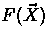to the following generalized form (or equivalent):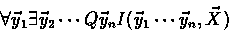(Q is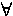if n is odd and is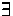otherwise.) where I is an arithmetic expression and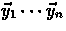are either explicitly bounded or their bounds can be resolved by context.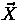is composed of local variables or exported variables from other process instances. Without loss of generality, assumeare exactly those variables which can not be resolved to deterministic value (otherwise we just hide them away). Furthermore, we restrict an Exit assertion to the following form: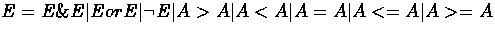The atomic assertion A in the formula above is defined by,

A=X|C|A+A|A-A

where

C=P.X|n|X'

In the formula above, C holds all deterministic values including exported variables P.X from another process P, constant expression n , and X' (the value that holds for X before starting the transition). All the other variablesare supposed to be changed after the transition. Without loss of generality, let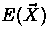stand for any Exit assertion as above. Define the Boolean structure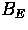as follows: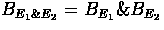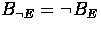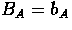where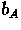is a Boolean variable indexed by A . Letdenote all Boolean variables inand define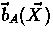, an assignment ofaccording to, as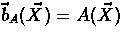.

Example.


EXIT
((X=Y+1) or (Y=Z)) & X>Z


whose Boolean structure is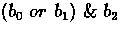For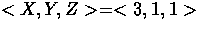, thewill be.

Say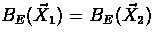if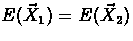and.

Denote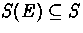to be the solution space ofwith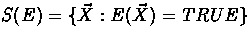.

Define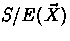to be a partition of S(E) :

(1).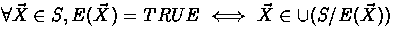,

(2).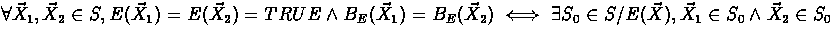.

THEOREM.is finite.

Let S(F) be the solution space of F .

THEOREM.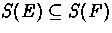is undecidable.

Based upon these theorems, the best thing we can do with the model checker is to check F by guessing the solution as follows:

Let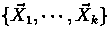be samples chosen from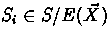. Check F with these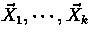respectively. F may be satisfied by these values but it may fail with other possible values. Because of the undecidability, in general we can't detect this failure. Hence by this algorithm, each transition branches into finite successors.Next: Analysis of cryptographic protocols Up: Using the ASTRAL Model Previous: Overview of ASTRAL and